8th Grade Math Home > Teacher Resources > Activities > Bungee m&ms: Student Worksheet

Bungee m&m’s: Student Worksheet

Name: ____________________________________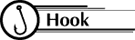Several years ago amusement parks introduced the thrill of bungee jumping to their guests. The basic idea of bungee jumping was to jump from a great height with an elastic cord tied to oneself so as to bounce up and down from the end of the cord.

The need for advanced planning and testing of this apparatus is obvious. Modeling the situation is a much safer means of determining safety factors than making actual trials and perhaps fatal errors!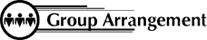Students work in pairs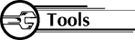Each pair needs:

• 1 bungee cord (plastic Slinky spring)
• 2 meter sticks
• 30 m&m’s
• 2 paperclips
• 1 paper cup1. Collect the Data
1. Place the meter stick level between two desks
2. Using the paper clips, attach the cup to one end of the spring.
3. Hook the other end of the spring to the meter stick so that it hangs evenly between the two desks.
4.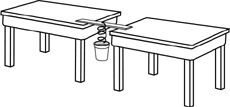5. Measure the distance from the bottom of the cup to the floor with zero m&m’s in the cup.
6. Record the distance in the table below.
7. Add one m&m to the cup.
8. After the cup comes to a standstill, measure the distance to the floor again.
9. Record the distance in the table below.
10. Repeat steps 6 through 8 four more times, adding one more m&m each time and recording the data in the table below.

 Weight (# m&m's) Independent Variable X Distance to Floor (cm) Dependent Variable Y 0 1 2 3 4 5

2. Graph the Data
1. Graph your ordered pairs on a coordinate plane with the Weight (# of m&m’s) on the horizontal axis and the Distance (cm) on the vertical axis. Be sure to label both axes and give your graph a title. When deciding what units to put on your axes, keep in mind that you will need to be able to use your graph to predict for shorter distances and as many as 30 m&m’s.

3. Analyze the Data
1. Look at your graphed points. What kind of correlation does this graph have? How can you tell?
2. As you add weight to the Bungee Cup, what happens to the distance to the floor?
3. By about how many centimeters does the distance to the floor decrease every time you increase the weight by one m&m?

4. Draw the Line of Best Fit
1. Look at your graphed points. Do they appear to lie along a straight line or a curve?
2. Use a straight edge to draw a "line of best fit" through the set of points you have graphed. Be sure it passes through at least 2 of your data points, leaving about the same number of other points on each side of the "line of best fit." Be sure to extend the line as far as possible!
3. What was the distance (cm) to the floor before any weight was added to the cup? How can you tell this from your graph?
4. How does that information relate to the "line of best fit" on your graph?
5. Describe in words how to determine the distance from the floor (y) if you know the weight (x).

5. Make Predictions
1. Use your graph with the "line of best fit" and the data in your table to predict how many m&m’s you will be able to put into the cup so that the bungee cup comes as close to the floor as possible without hitting the floor.

Total number of m&ms

Check your prediction . . . . .BE CAREFUL!!!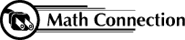As a result of this activity, students learn to model a real-life situation by collecting data from a few trials, graphing the data, and then drawing a line of best fit that can be used to make predictions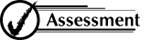1. Using the two points that connected the "line of best fit," calculate the slope of your line.

(   ,   ) (   ,   )
slope: _______
m =
_______

2. Write the equation for your "line of best fit" in slope-intercept form:

y = ______

 8th Grade Math Home > Teacher Resources > Activities > Bungee m&ms: Student Worksheet